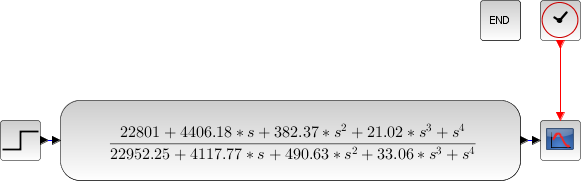Change language to:
English - Français - 日本語 - Português -

See the recommended documentation of this function

Справка Scilab >> Xcos > Batch functions > xcos_simulate

# xcos_simulate

Function for running xcos simulation in batch mode

### Syntax

`[Info, status]=xcos_simulate(scs_m, needcompile)`

### Arguments

scs_m: A diagram data structure (see scs_m structure).

needcompile: An integer. If it equals 4, then the simulator will perform a complete compilation of the diagram. If not, it will use cached value of %cpr. DEPRECATED
status: A boolean. %T if simulation is successful, %f if simulation fails.

### Description

This function is used to simulate xcos diagrams in batch mode. It requires the scs_m structure which can be obtained by loading in Scilab the `.zcos` file (see importXcosDiagram ).

User can also define `pre_xcos_simulate` function that will run before simulation.

```function continueSimulation=pre_xcos_simulate(scs_m, needcompile)
// Do nothing
continueSimulation = %t;
endfunction```
If this function fails the error will be trapped and the simulation aborted. If `continueSimulation` is set to false, the simulation will stop.

User can also define `post_xcos_simulate` function that will run after simulation.

```function post_xcos_simulate(%cpr, scs_m, needcompile)
// Do Nothing
endfunction```
If this function fails the error will be trapped.

### File content

• SCI/modules/xcos/macros/xcos_simulate.sci

### Examples

The xcos diagram in SCI/modules/xcos/demos/batch_simulation.zcos.Simulate this diagram in batch mode and change some context values.
```importXcosDiagram("SCI/modules/xcos/demos/batch_simulation.zcos")

typeof(scs_m) //The diagram data structure

//This diagram uses 3 context variables :
//  Amplitude : the sin function amplitude
//  Pulsation : the sin function pulsation
//  Tf        : the final simulation time
scs_m.props.context //the embedded definition

//first batch simulation with the parameters embedded in the diagram
xcos_simulate(scs_m, 4);

// Change the final time value
scs_m.props.context = ["Amplitude=2" "Pulsation=3" "Tf=10"];
xcos_simulate(scs_m, 4);

// Now Change pulsation
scs_m.props.context = ["Amplitude=2" "Pulsation=9" "Tf=10"];
xcos_simulate(scs_m, 4);
//get the variable created by the "from workspace block"
counter```

Statistic analysis of the diagram using pre_xcos_simulate function.

```importXcosDiagram("SCI/modules/xcos/demos/batch_simulation.zcos")

typeof(scs_m) //The diagram data structure

// Declare pre_xcos_simulation to only analyze Diagram.

function continueSimulation=pre_xcos_simulate(scs_m, needcompile)
// Retrieve all objects
objs = scs_m.objs;

blocks = 0;
other = 0;
for i = 1:size(objs)
currentType = typeof(objs(i));
select (currentType)
case "Block"
blocks = blocks + 1;
else
other = other + 1;
end
end

// Diplay Diagram analisys.
disp("Diagram Analysis:")
disp("Found "+string(blocks)+" Blocks.")
disp("Found "+string(other)+" Other component.")

// We do not want any simulation, just analyze Diagram
continueSimulation = %f;
endfunction

//first batch simulation with the parameters embedded in the diagram
xcos_simulate(scs_m, 4);```

The xcos diagram: SCI/modules/xcos/demos/Command.zcosSimulate this diagram in batch mode and perform bode plotting of transfert function using post_xcos_simulate,
```importXcosDiagram("SCI/modules/xcos/demos/Command.zcos")

// Clear any pre_xcos_simulation;
clear pre_xcos_simulate;

function post_xcos_simulate(%cpr, scs_m, needcompile)
// Retrieve all objects
objs = scs_m.objs;

clrBlock = [];
//Looking for CLR block
for i=1:size(objs)
if objs(i).gui == "CLR" then
clrBlock = objs(i);
break;
end
end

// Check if we found some CLR
if isempty(clrBlock) then
disp("No CLR block found.")
return
end

// Retrieve exprs
exprs = clrBlock.graphics.exprs;
s = poly(0,'s');
num = evstr(exprs(1));
den = evstr(exprs(2));
h = syslin('c', num/den);
// Open new figure then plot Bode
scf(max(winsid())+1);
bode(h, 0.01, 100);
endfunction

xcos_simulate(scs_m, 4);```

The xcos diagram: SCI/modules/xcos/demos/Command_bode.zcosSimulate this diagram in batch mode and perform bode plotting of transfert function using post_xcos_simulate,
```importXcosDiagram("SCI/modules/xcos/demos/Command_bode.zcos")

// Clear any post_xcos_simulation;
clear post_xcos_simulate;

function continueSimulation=pre_xcos_simulate(scs_m, needcompile)
// Retrieve all objects
objs = scs_m.objs;

clrBlock = [];
//Looking for CLR block
for i=1:size(objs)
if objs(i).gui == "CLR" then
clrBlock = objs(i);
break;
end
end

// Check if we found some CLR
if isempty(clrBlock) then
disp("No CLR block found.")
return
end

// Retrieve exprs
exprs = clrBlock.graphics.exprs;
s = poly(0,'s');
num = evstr(exprs(1));
den = evstr(exprs(2));
h = syslin('c', num/den);
// Open new figure then plot Bode
scf(max(winsid())+1);
bode(h, 0.01, 100);

// Stop after bode plot. We do not want any simulation.
continueSimulation = %f;
endfunction

xcos_simulate(scs_m, 4);```